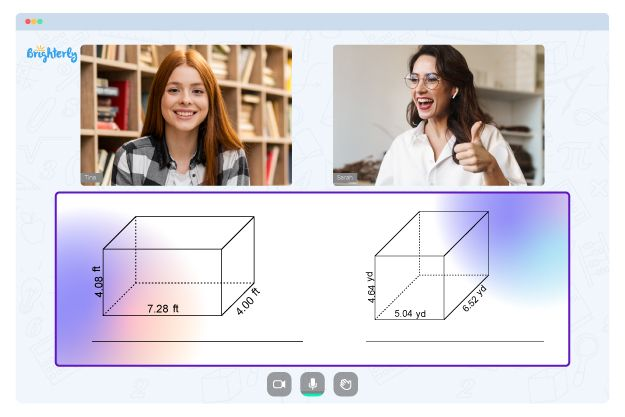# Special Parallelograms Worksheets

A rhombus, rectangle, and square are regarded as special parallelograms because they fit the descriptions of equal angles and lengths on opposite sites. However, because they have unique properties, they are called special parallelograms. For kids to understand special parallelograms better, they need a special parallelograms worksheet.

• A square is a parallelogram with sides equal in length and similar in angle.
• A rectangle is a parallelogram where all sides give you 90 degrees (90°).
• A Rhombus has four matching sides and is also called a diamond.

## The benefits of using the special parallelograms worksheet

Math for Kids

Is Your Child Struggling With Math?
1:1 Online Math TutoringThe special parallelograms worksheet answers benefit students in many ways. Most importantly, it helps kids understand the different shapes in the parallelogram universe.

Understanding special parallelograms involves learning about these shapes in their individuality. Using the parallelograms, rectangles, rhombi, and squares worksheet can help your kid identify these shapes and understand their peculiarity. They also figure out how and how the shapes fit into the parallelogram conversation. You can use the rectangles, rhombi, and squares worksheet to teach kids all they need to learn about special parallelograms.### Special Parallelograms Worksheets PDF

Special Parallelograms Worksheet### Special Parallelograms Worksheets PDF### Special Parallelograms Worksheets PDF

Parallelograms Rectangles Rhombi And Squares Worksheet### Special Parallelograms Worksheets PDF

Rectangles Rhombi And Squares Worksheet

Problems with Geometry?• Does your child struggle with understanding geometry concepts?
• Try learning with an online tutor.

Is your child having trouble mastering of geometry? An online tutor could provide the necessary assistance.